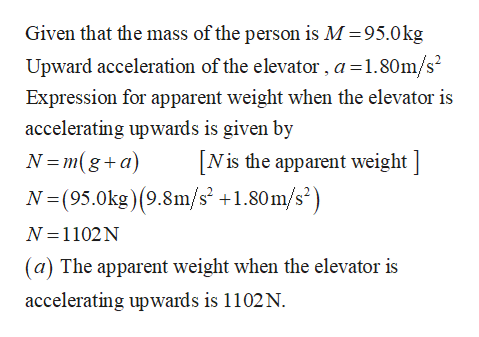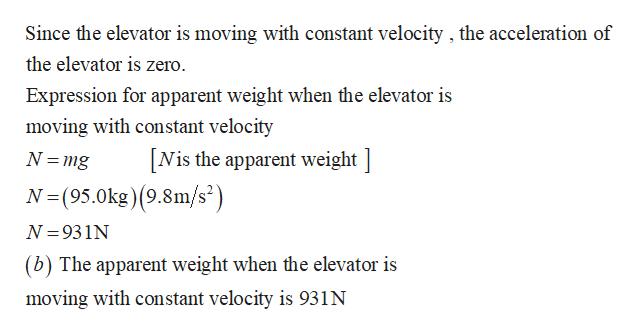# A 95.0 kg person stands on a scale in an elevator. What is the "apparent weight" when the elevator is:a. accelerating upwards with an acceleration of 1.80 m/s^2b. moving upward at a constant speedc. accelerating downwards with an acceleration of 1.30 m/s^2

Question
11 views

A 95.0 kg person stands on a scale in an elevator. What is the "apparent weight" when the elevator is:

a. accelerating upwards with an acceleration of 1.80 m/s^2

b. moving upward at a constant speed

c. accelerating downwards with an acceleration of 1.30 m/s^2

check_circle

Step 1

Write the expression for apparent weight when the elevator is accelerating upwards.help_outlineImage TranscriptioncloseGiven that the mass of the person is M =95.0kg Upward acceleration of the elevator , a =1.80m/s? Expression for apparent weight when the elevator is accelerating upwards is given by N =m(g+a) [Nis the apparent weight ] N=(95.0kg)(9.8m/s² +1.80m/s²) N =1102N (a) The apparent weight when the elevator is accelerating upwards is 1102 N. fullscreen
Step 2help_outlineImage TranscriptioncloseSince the elevator is moving with constant velocity , the acceleration of the elevator is zero. Expression for apparent weight when the elevator is moving with constant velocity [Nis the apparent weight ] N =mg N=(95.0kg)(9.8m/s³) N = 931N (b) The apparent weight when the elevator is moving with constant velocity is 931N fullscreen

### Want to see the full answer?

See Solution

#### Want to see this answer and more?

Solutions are written by subject experts who are available 24/7. Questions are typically answered within 1 hour.*

See Solution
*Response times may vary by subject and question.
Tagged in

### Newtons Laws of Motion